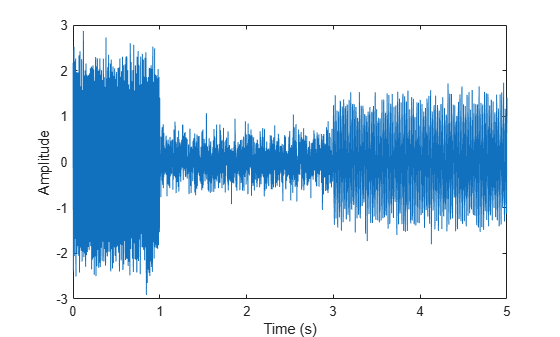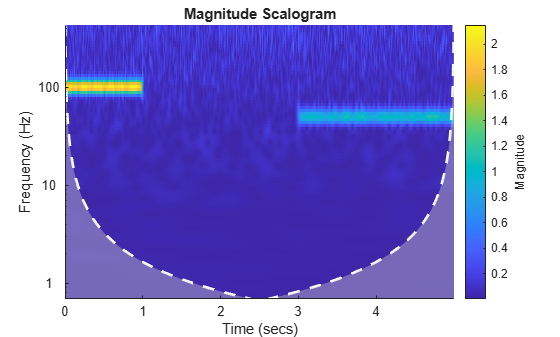# Continuous Wavelet Analysis

This example shows how to perform time-frequency analysis using the continuous wavelet transform (CWT). Continuous wavelet analysis provides a time-scale/time-frequency analysis of signals and images. The Wavelet Toolbox™ software has both command line and interactive functionality to support continuous wavelet analysis of 1-D signals.

Construct a signal consisting of two sinusoids with frequencies of 100 and 50 Hz, and white noise. The support of the two sinusoids is disjoint. The 100-Hz sine wave begins at t = 0 and has a duration of 1 second. The 100-Hz sinusoid has an amplitude of 2. The 50-Hz sinusoid begins at three seconds and has a duration of two seconds. The 50-Hz sinusoid has an amplitude of 1. The sampling frequency is 1 kHz. The signal length is 5000 samples.

```Fs = 1000; t = linspace(0,5,5e3); x = 2*cos(2*pi*100*t).*(t<1)+cos(2*pi*50*t).*(3<t)+0.3*randn(size(t));```

Plot the signal.

```plot(t,x) xlabel('Time (s)') ylabel('Amplitude')```Use `cwt` to obtain the CWT of the signal and plot its scalogram. The magnitudes of the sinusoid components in the colorbar are essentially their amplitudes even though they are at different scales.

`cwt(x,Fs)`